## research papersJOURNAL OFSYNCHROTRONRADIATION
ISSN: 1600-5775
Volume 15| Part 5| September 2008| Pages 514-518

## On the Einstein model for EXAFS parallel and perpendicular mean-square relative displacements

aUniversità degli Studi di Verona, Dipartimento di Informatica, Strada le Grazie 15, I-37134 Verona, Italy
*Correspondence e-mail: sanson@sci.univr.it

(Received 14 March 2008; accepted 11 June 2008; online 22 July 2008)

The correlated Einstein model for EXAFS parallel and perpendicular mean-square relative displacements (MSRDs) is discussed. By means of dynamical simulations on different crystalline structures, the error owing to the Einstein-fit model on the EXAFS MSRDs is estimated as a function of the standard deviation of the density of vibrational states. This error should be taken into account to improve the accuracy of the MSRDs.

### 1. Introduction

Extended X-ray absorption fine structure (EXAFS) is known as a powerful probe for investigating disordered systems and, thanks to accurate temperature-dependent measurements, for obtaining original information on the local dynamics of crystals, such as the parallel and perpendicular mean-square relative displacements (Brewe et al., 1997; Fornasini et al., 2004). Indicating by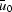and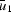the thermal displacement of the absorber and backscatterer atoms of EXAFS, respectively, the parallel mean-square relative displacement (MSRD) is defined as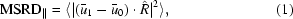and the perpendicular mean-square relative displacement (MSRD) as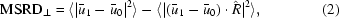where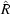is the unit vector of the equilibrium distance between the two atoms. The EXAFS Debye–Waller factor directly gives information on MSRD (Beni & Platzman, 1976). The difference between the interatomic distance 〈r〉 = 〈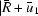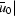〉 probed by EXAFS and the crystallographic distance R =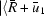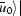between average positions allows measurement of the temperature dependence of MSRD (Dalba et al., 1999).

The EXAFS MSRDs represent a test bench for lattice dynamical theories; their comparison with the absolute mean-square displacements (MSDs) obtained from X-ray diffraction allows evaluation of the correlation of atomic motions, which plays a key role in the study of some physical phenomena such as phase transitions and negative thermal expansion (Sanson et al., 2006).

To find accurate values of MSRD and MSRD by EXAFS, an accurate knowledge of the EXAFS scattering amplitudes, phase shifts and inelastic terms is required (Teo, 1986). These parameters can be determined by theoretical calculations, for example using FEFF8 code (Ankudinov et al., 1998). However, the uncertainties on these calculations affect the accuracy on the absolute value of MSRD and MSRD. An alternative procedure consists of a separate analysis of phase and amplitude of the filtered EXAFS signal via the ratio method (Bunker, 1983; Tranquada & Ingalls, 1983) taking the lowest-temperature spectra as reference for backscattering amplitudes, phase shifts and inelastic terms. However, only the relative differences δMSRD(T) = MSRD(T) − MSRD(T0) and δMSRD(T) = MSRD(T) − MSRD(T0) with respect to the reference temperature T0 can be obtained from this second procedure. In both procedures it is customary to fit the temperature dependence of δMSRD(T) and δMSRD(T) to a correlated Einstein model in order to obtain with reasonable accuracy the absolute value of MSRD(T) and MSRD(T) (Dalba & Fornasini, 1997).

In the correlated Einstein model, which consists of substituting the phonon density of states ρ(ν) with a delta function centred at νE, one obtains the following expressions for MSRD(T) and MSRD(T) (Sevillano et al., 1979; Vaccari & Fornasini, 2006),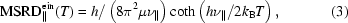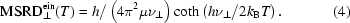To obtain the absolute values of MSRD(T) and MSRD(T) from the experimental temperature-dependence of δMSRD(T) and δMSRD(T), one uses the following fit expressions,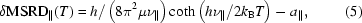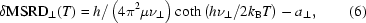where a, a and the Einstein frequencies ν, ν are the only fitting parameters. The absolute values of MSRD(T) and MSRD(T) are obtained, respectively, by shift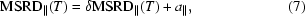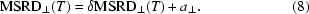Although the Einstein model is based on a strong assumption (phonon spectrum approximated to a single frequency), it is a matter of experience that the temperature dependence of the experimental MSRDs can be reasonably fitted by this model. However, the error deriving from this procedure has never been estimated. This is the aim of this work: to estimate, for the first time, the error on the absolute value of the EXAFS MSRDs owing to the use of the Einstein-fit model.

The paper is organized as follows: in §2the procedure of estimating the EXAFS MSRDs error is briefly described; in §3the results are reported and discussed; a remark on the connection between Einstein-model and crystal dynamics is made in §4; §5is dedicated to conclusions.

### 2. Procedure

As a first step, the temperature dependences of MSRD(T) and MSRD(T) have been calculated (between 0 and 1000 K) in different crystals by means of dynamical simulations. The dynamical calculations have been performed within the Born–Von Karman approximation (Maradudin et al., 1971) on the following crystalline structures: face-centered cubic, diamond structure, wurtzite and cuprite (one, two, four and six atoms in the elementary cell, respectively) with arbitrary force constants and atomic masses, in all 27 crystals. The parallel and the perpendicular MSRD(T) are calculated in terms of eigenfrequencies ν(λ,) and eigenvectors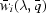of the dynamical matrix (for more details see Maradudin et al., 1971; Vaccari & Fornasini, 2006) as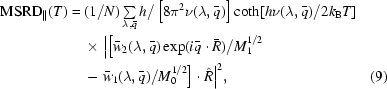and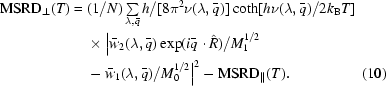As a second step, the relative differences with respect to 0 K, i.e. δMSRD(T) = MSRD(T) − MSRD(0) and δMSRD(T) = MSRD(T) − MSRD(0), have been fitted with the correlated Einstein model in agreement with equations (5)and (6), respectively. As a result, the fitting parameters a and a (as well as ν and ν) have been obtained for each crystal.

For the third step, if the Einstein-fit error is zero, then a = MSRD(0) and a = MSRD(0), and so δMSRD(T) + a and δMSRD(T) + a must give MSRD(T) and MSRD(T), respectively. As a consequence, the percentage errors on MSRD and MSRD at temperature T have been estimated, respectively, as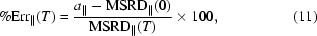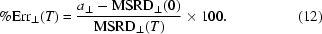Fig. 1shows an example of calculated MSRD(T), the relative difference δMSRD(T) with respect to 0 K, and the corresponding Einstein fit by equation (5). The resulting shift between MSRD(T) and δMSRD(T) + a (open and full circles, respectively) is the error on the absolute MSRD(T) owing to the Einstein fit.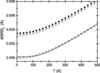Figure 1 Example of the Einstein fit on the parallel mean-square relative displacement: MSRD∥(T) calculated by dynamical simulation (open circles), relative differences δMSRD∥(T) with respect to 0 K (open squares) and corresponding Einstein fit (line). The resulting MSRD∥(T) from the Einstein fit (full circles) is shifted with respect to the correct MSRD∥(T) (open circles).

It can be expected that the error owing to the Einstein fit increases with an increase in the standard deviation of the density of vibrational states (DOS) of the crystal. In a `perfect Einstein crystal', where DOS is a δ function and the DOS standard deviation is zero, the Einstein-fit error is zero. As a consequence, in §3, for each crystal the Einstein-fit error has been plotted against the DOS standard deviation. Fig. 2shows three examples of the calculated DOS with different standard deviations.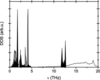Figure 2 Examples of calculated phonon DOS with different standard deviations: 1.3 THz (gray), 3.0 THz (white) and 4.8 THz (black).

### 3. Results and discussion

According to equations (5)–(6)and (9)–(12), the percentage errors on MSRD(T) and MSRD(T) at 0, 300 and 600 K have been calculated and plotted against the DOS standard deviation of the crystal (Figs. 3and 4). The Einstein fit has been performed over a typical experimental range, between 0 and 500 K. To first approximation, the correlation between the Einstein error and the DOS standard deviation is independent of the crystal structure.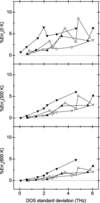Figure 3 Percentage error given by the Einstein fit on the absolute MSRD∥(T) at 0, 300 and 600 K, calculated in different crystalline structures: face-centered cubic (full-down triangles), diamond (open-down triangles), wurtzite (full-up triangles) and cuprite (open-up triangles). Lines are a guide for the eyes.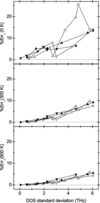Figure 4 Percentage error given by the Einstein fit on the absolute MSRD⊥(T) at 0, 300 and 600 K. Symbols are the same as in Fig. 3.

From Figs. 3and 4it can be observed that (i) the Einstein fit gives an overestimation of both MSRD(T) and MSRD(T) (%Err and %Err are always greater than zero); (ii) the error on MSRD(T) is about two times the error on MSRD(T) (%Err ≃ 2%Err); (iii) on average, the error is within 5% on MSRD(T) and within 10% on MSRD(T), at least if 0–500 K is considered as the fitting interval.

It is obvious that the error owing to the Einstein fit depends on the fitting interval. Since at high temperatures equations (5)–(6)are approximated by a classical linear behaviour, problems are mainly expected at low temperatures. Fig. 5reports the percentage error in the diamond structure obtained with four different fitting intervals: 0–300 K, 0–500 K, 0–1000 K and 200–1000 K. The error is reduced when the high-temperature range prevails against the low-temperature range in the fitting procedure. Analogous results have been obtained for the other three crystalline structures.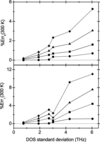Figure 5 Percentage error given by the Einstein fit on the absolute MSRD∥(T) and MSRD⊥(T) (top and bottom panel, respectively) in diamond structure at 300 K for different fitting intervals: 0–300 K (diamonds), 0–500 K (triangles), 0–1000 K (squares) and 200–1000 K (circles). The error reduces when the high temperatures prevail in the fitting range.

From this result one finds that it is more convenient to fit the experimental δMSRD(T) and δMSRD(T) neglecting the low-temperature intervals. However, this is true only in ideal situations. In real situations, where δMSRD(T) and δMSRD(T) are affected by experimental uncertainties, it is probably more convenient to fit the whole available temperature range in order to maximize the experimental information. In any case, the high-temperature ranges reduce the Einstein error on the absolute MSRDs.

Only recently expression (4)for perpendicular MSRD has been derived by Vaccari & Fornasini (2006). In previous works (for example, Dalba et al., 1999; Fornasini et al., 2004) the absolute MSRD(T) was estimated from the correlated Einstein model for parallel MSRD, i.e. using equation (5)instead of equation (6). The use of equation (5), which differs by a factor of two from equation (6), is equivalent to inserting an incorrect value of the reduced mass μ (twice the real value) in the fitting procedure of MSRD(T). I have estimated the error owing to the utilization of equation (5)instead of equation (6): as shown in Fig. 6, equation (5)gives an underestimation (%Err < 0) on the absolute value of MSRD(T), and the error is about twice that resulting from equation (6). As a consequence, equation (6)is fundamental in the fitting of perpendicular MSRD. For the first time it has been used by Vaccari et al. (2007) on cuprous chloride.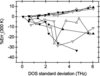Figure 6 Percentage error on the absolute MSRD⊥(T) at 300 K: with δMSRD⊥(T) fitted by equation (5)(negative values) and by equation (6)(positive values). The fit was made between 0 and 500 K. Symbols are the same as in Fig. 3.

In the practical EXAFS analysis the present results allow a first estimation to be given of the error on the absolute MSRDs, simply from the DOS standard deviation of the crystal. Typical values of the DOS standard deviation are of the order of a few THz. As an example, let us consider the case of crystalline germanium, which displays a DOS standard deviation of about 3 THz (Bruesch, 1982). From Figs. 3and 4, the Einstein fit between 0 and 500 K overestimates MSRD by about 2% at 300 K, and MSRD by about 3%. In the case of the Einstein fit performed between 0 and 300 K, the error increases by about 1.5 times (as evident by Fig. 5), and the overestimation on MSRD and MSRD increases by about 3% and 5%, respectively. Accordingly, the resulting EXAFS MSRDs should be properly corrected to improve their accuracy.

### 4. Einstein model and crystal dynamics

Before the conclusion, let us make a final consideration on the Einstein model and on the corresponding crystal dynamics.

Let us indicate by γ(T) the ratio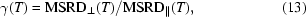which measures the anisotropy of relative thermal vibrations. If MSRD(T) and MSRD(T) can be described by a correlated Einstein model, then by inserting equations (3)and (4)into equation (13)one obtains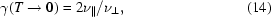and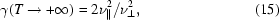and, by comparing the last two equations,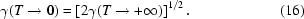As a result, in the correlated Einstein model the temperature dependence of γ(T) follows the condition expressed by equation (16). This is the case, for example, of perfect isotropy, where γ(T) = 2 at all temperatures.

Fig. 7shows the ratio [2γ(T → +∞)]1/2/γ(T → 0) calculated in the 27 crystals of this work [with γ(T → +∞) approximated to γ at 1000 K]: in most cases this ratio is closer to unity. However, this does not mean that the Einstein model works well: equation (16)is a necessary but not sufficient condition of the crystal dynamics to describe MSRD(T) and MSRD(T) by the correlated Einstein model.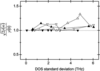Figure 7 Calculated ratio [2γ(T → +∞)]1/2/γ(T → 0). Symbols are the same as in Fig. 3.

### 5. Conclusions

In this work the error owing to the Einstein fit on the absolute value of EXAFS MSRDs has been estimated by means of dynamical simulations. On average the error is less than ∼5% for the parallel MSRD, less than 10% for the perpendicular MSRD, but it should be taken into account to improve the MSRDs accuracy. The fitting error depends on the fitting range and, in principle, decreases neglecting the low temperatures. Finally, in the fitting of perpendicular MSRD, the importance of equation (6)has been demonstrated.

### Acknowledgements

I would like to thank P. Fornasini and M. Vaccari for helpful discussions.

### ReferencesJOURNAL OFSYNCHROTRONRADIATION